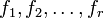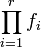# Schinzel's hypothesis H

Supposeare all irreducible polynomials with integer coefficients and with positive leading coefficient, such that the productdoes not have any fixed divisors, i.e., it cannot be expressed as a proper multiple of an integer-valued polynomial. Schinzel's hypothesis H states that there are infinitely many natural numberssatisfying the condition thatare all simultaneously prime.
Twin prime conjecture open We are dealing with the irreducible polynomialsand